$$\gdef\badDispatch#1{\textbf{\textcolor{e1432d}{#1}}} \gdef\noKatexForm#1{\badDispatch{#1}} \gdef\largeDot{\raisebox{0.06em}{\tiny∙}} \gdef\rarrbar{ {\raisebox{-0.05em}{→}\mkern{-0.13em}{\large\shortmid}}} \gdef\larrbar{ { {\large\shortmid}\mkern{-0.13em}{\raisebox{-0.05em}{←}}}} \gdef\suptrans{^\mathsf{T}} \gdef\supdagger{^\dagger} \gdef\rawsymbol#1{\operatorname{#1}} \gdef\colorsymbol#1#2{\textcolor{#1}{\rawsymbol{#2}}} \gdef\lsymbol#1{\colorsymbol{262626}{#1}} \gdef\msymbol#1{\colorsymbol{b50800}{#1}} \gdef\osymbol#1{\colorsymbol{00427f}{#1}} \gdef\lstring#1{\textcolor{666666}{\textrm{\textquotedblleft{\text{#1}}\textquotedblright}}} \gdef\boldForm#1{\textbf{#1}} \gdef\lrform#1{ {\textcolor{e1432d}{#1}}} \gdef\lgform#1{ {\textcolor{4ea82a}{#1}}} \gdef\lbform#1{ {\textcolor{3e81c3}{#1}}} \gdef\lrgform#1{ {\textcolor{ffc87d}{#1}}} \gdef\lgbform#1{ {\textcolor{96e2e3}{#1}}} \gdef\lrbform#1{ {\textcolor{f989b8}{#1}}} \gdef\rform#1{ {\textcolor{cb2b1a}{#1}}} \gdef\gform#1{ {\textcolor{399318}{#1}}} \gdef\bform#1{ {\textcolor{2b6fb0}{#1}}} \gdef\drform#1{ {\textcolor{9b0000}{#1}}} \gdef\dgform#1{ {\textcolor{006800}{#1}}} \gdef\dbform#1{ {\textcolor{004987}{#1}}} \gdef\drgform#1{ {\textcolor{ae5900}{#1}}} \gdef\dgbform#1{ {\textcolor{006567}{#1}}} \gdef\drbform#1{ {\textcolor{86004d}{#1}}} \gdef\rgform#1{ {\textcolor{dc841a}{#1}}} \gdef\gbform#1{ {\textcolor{47a5a7}{#1}}} \gdef\rbform#1{ {\textcolor{c74883}{#1}}} \gdef\pform#1{ {\textcolor{665996}{#1}}} \gdef\dpform#1{ {\textcolor{41326b}{#1}}} \gdef\waform#1{ {\textcolor{6b6b6b}{#1}}} \gdef\wbform#1{ {\textcolor{929292}{#1}}} \gdef\wcform#1{ {\textcolor{c5c5c5}{#1}}} \gdef\tick{\checkmark} \gdef\barToken{\mathbf{|}} \gdef\filledRectangleToken{▮} \gdef\emptyRectangleToken{▯} \gdef\filledSquareToken{■} \gdef\emptySquareToken{□} \gdef\filledToken{∙} \gdef\emptyToken{∘} \gdef\cardinalRewrite#1#2{\rewrite{#1}{#2}} \gdef\primed#1{ {#1}^{\prime}} \gdef\tinybullet{ {\tiny●}} \gdef\factsym#1{\mathord{#1}} \gdef\forwardFactor{\factsym{\uparrow}} \gdef\backwardFactor{\factsym{\downarrow}} \gdef\forwardBackwardFactor{\factsym{\updownarrow}} \gdef\forwardBackwardNeutralFactor{\factsym{\mathrlap{\downarrow}{\mathrlap{\uparrow}{\endash} } }} \gdef\neutralFactor{\factsym{\shornarrow}} \gdef\arrowhead{⏵} \gdef\inverseArrowhead{⏴} \gdef\rightArrowhead{⏵} \gdef\leftArrowhead{⏴} \gdef\upArrowhead{⏶} \gdef\downArrowhead{⏷} \gdef\de#1#2{ { {#1}\xundirectededge{}{#2}}} \gdef\ue#1#2{ { {#1}\xrightedge{}{#2}}} \gdef\shifttag#1{\raisebox{-1em}{#1}} \gdef\shifttag#1{#1} \gdef\tde#1#2#3{ {#1}\:\xrightedge{\shifttag{#3}}\;{#2}} \gdef\tue#1#2#3{ {#1}\;\xundirectededge{\shifttag{#3}}\;{#2}} \gdef\shiftunderset#1#2{\underset{\raisebox{0.15em}{\scriptsize #1}}{#2}} \gdef\mdtde#1#2#3#4{ {#1}\,\;\shiftunderset{#4}{\xrightedge{#3}}\;{#2}} \gdef\mtue#1#2#3#4{ {#1}\,\;\shiftunderset{#4}{\xundirectededge{#3}}\;\,{#2}} \gdef\mtde#1#2#3#4{ {#1}\,\;\operatornamewithlimits{\xrightedge{#3}}\limits_{#4} \;{#2}} \gdef\mapsfrom{\htmlClass{hreflect}{\mapsto}} \gdef\longmapsfrom{\htmlClass{hreflect}{\longmapsto}} \gdef\diffd{𝕕} \gdef\partialdof#1{\partial {#1}} \gdef\emptySet{\empty} \gdef\unknown{\wbform{\text{?}}} \gdef\notApplicable{\wbform{\text{---}}} \gdef\textAnd{\text{\,and\,}} \gdef\identicallyEqualSymbol{\equiv} \gdef\congruentSymbol{\equiv} \gdef\isomorphicSymbol{\simeq} \gdef\homeomorphicSymbol{\cong} \gdef\homotopicSymbol{\simeq} \gdef\approxEqualSymbol{\approx} \gdef\bijectiveSymbol{\approx} \gdef\defeq{\mathrel{≝}} \gdef\defEqualSymbol{\mathrel{≝}} \gdef\syntaxEqualSymbol{\mathrel{\textcolor{888888}{\equiv}}} \gdef\tailEqualSymbol{\underdot{=}} \gdef\headEqualSymbol{\dot{=}} \gdef\ruledelayed{:\to} \gdef\mathdollar{\text{\textdollar}} \gdef\hyphen{\text{-}} \gdef\endash{\text{--}} \gdef\emdash{\text{---}} \gdef\updownarrows{\uparrow\!\downarrow} \gdef\vthinspace{\mkern{1pt}} \gdef\dlq{\text{\textquotedblleft}} \gdef\drq{\text{\textquotedblright}} \gdef\dprime{ {\prime\prime}} \gdef\inverse#1{ { {#1}^{-1}}} \gdef\inverseSymbol{\inverse{□}} \gdef\groupDirectProduct#1{#1} \gdef\groupDirectProductSymbol{\times} \gdef\groupInverse{\inverse} \gdef\groupPower#1#2{ {#1}^{#2}} \gdef\groupCommutator#1#2{\left[{#1},{#2}\right]} \gdef\groupoidInverse{\inverse} \gdef\latticeBFS#1{\textrm{bfs}({#1})} \gdef\pathHomomorphism#1{#1} \gdef\groupoidFunction#1{#1} \gdef\groupoidHomomorphism#1{#1} \gdef\affineModifier#1{\overrightharpoon{#1}} \gdef\supercirc#1{#1^{\circ}} \gdef\supercircb#1{#1^{\bullet}} \gdef\blackCircleModifier#1{\supercircb{#1}} \gdef\whiteCircleModifier#1{\supercirc{#1}} \gdef\totalSpaceStyle#1{\rbform{#1}} \gdef\baseSpaceStyle#1{\bform{#1}} \gdef\fiberSpaceStyle#1{\rform{#1}} \gdef\totalSpaceElementStyle#1{\drbform{#1}} \gdef\baseSpaceElementStyle#1{\dbform{#1}} \gdef\fiberSpaceElementStyle#1{\drform{#1}} \gdef\bundleProjectionStyle#1{\dgform{#1}} \gdef\bundleGraphStyle#1{\rbform{#1}} \gdef\bundleSectionStyle#1{\drgform{#1}} \gdef\bundleFunctionStyle#1{\drbform{#1}} \gdef\graphFunctionStyle#1{\totalSpaceStyle{#1}} \gdef\projectionFunctionStyle#1{\totalSpaceStyle{#1}} \gdef\sectionFunctionStyle#1{\totalSpaceStyle{#1}} \gdef\functionGraph#1{G_{#1}} \gdef\toroidalModifier#1{\supercirc{#1}} \gdef\modulo#1{\supercirc{#1}} \gdef\dividesSymbol{\mathrel{|}} \gdef\groupFunction#1{#1} \gdef\groupHomomorphism#1{#1} \gdef\automorphisms{\operatorname{Aut}} \gdef\endomorphisms{\operatorname{End}} \gdef\transportMap#1{\transportMapSymbol_{#1}} \gdef\transportMapSymbol{\tau} \gdef\action#1{#1} \gdef\selfAction#1{\hat{#1}} \gdef\actionGroupoid#1{\utilde{#1}} \gdef\cardinalGroup#1{G^*({#1})} \gdef\signed#1{ {#1}^*} \gdef\transportAtlas#1{T_{#1}} \gdef\inverted#1{\underline{#1}} \gdef\mirror#1{\overline{#1}} \gdef\pathComposeSymbol{\mathbin{∷}} \gdef\pathCompose#1#2{ {#1}\pathComposeSymbol{#2}} \gdef\translateSymbol{\mathbin{\uparrow}} \gdef\backwardTranslateSymbol{\mathbin{\downarrow}} \gdef\pathTranslate#1#2{ {#1}\translateSymbol{#2}} \gdef\pathLeftTranslate#1{ { {#1}\translateSymbol}} \gdef\pathBackwardTranslate#1#2{ {#1}{\backwardTranslateSymbol}{#2}} \gdef\pathLeftBackwardTranslate#1{ {#1}{\backwardTranslateSymbol}} \gdef\compactCovariantDifference#1#2{\Delta_{#1}\,{#2}} \gdef\covariantDifference{ {#1}\,\Delta\,{#2}} \gdef\forwardDifference{\Delta^{+}} \gdef\backwardDifference{\Delta^{-}} \gdef\centralDifference{\Delta} \gdef\pathForwardDifference#1{\forwardDifference_{#1}} \gdef\pathBackwardDifference#1{\backwardDifference_{#1}} \gdef\pathCentralDifference#1{\centralDifference_{#1}} \gdef\pathHead#1{\pathHeadVector{#1}} \gdef\pathTail#1{\pathTailVector{#1}} \gdef\pathHeadVector#1{ {#1}^{\bullet}} \gdef\pathTailVector#1{ {#1}_{\bullet}} \gdef\pathReverse#1{ {#1}^{\dagger}} \gdef\pathIntegral#1#2{ {#1} \int {#2}} \gdef\pathIntegralSymbol{ {\int}} \gdef\pathDot#1#2{ {#1} \cdot {#2}} \gdef\pathDotSymbol{ {\cdot}} \gdef\compactBasis#1{\mathscr{B}} \gdef\length{\operatorname{len}} \gdef\signedLength{\operatorname{len^*}} \gdef\andFn{\operatorname{and}} \gdef\orFn{\operatorname{or}} \gdef\notFn{\operatorname{not}} \gdef\vertexList{\operatorname{vertices}} \gdef\vertexList{\operatorname{vertices}} \gdef\edgeList{\operatorname{edges}} \gdef\pathList{\operatorname{paths}} \gdef\cardinalList{\operatorname{cards}} \gdef\signedCardinalList{\operatorname{cards^*}} \gdef\wordOf{\operatorname{word}} \gdef\headVertex{\operatorname{head}} \gdef\tailVertex{\operatorname{tail}} \gdef\basis{\operatorname{basis}} \gdef\split{\operatorname{split}} \gdef\lcm{\operatorname{lcm}} \gdef\minimalContractionSets{\operatorname{MCSets}} \gdef\minimalContractions{\operatorname{MC}} \gdef\grade{\operatorname{grade}} \gdef\support{\operatorname{support}} \gdef\coefficient{\operatorname{coeff}} \gdef\domain{\operatorname{domain}} \gdef\codomain{\operatorname{codomain}} \gdef\modFunction{\operatorname{mod}} \gdef\clip{\operatorname{clip}} \gdef\sign{\operatorname{sign}} \gdef\step{\operatorname{step}} \gdef\projection{\operatorname{proj}} \gdef\lift{\operatorname{lift}} \gdef\identity{\operatorname{id}} \gdef\total{\operatorname{total}} \gdef\torus{\operatorname{torus}} \gdef\mobius{\operatorname{mobius}} \gdef\stateCompose{\operatorname{glue}} \gdef\infixStateComposeSymbol{\_} \gdef\stateDecompose{\operatorname{melt}} \gdef\stateJoin{\operatorname{conj}} \gdef\stateMeet{\operatorname{disj}} \gdef\stateExtent{\operatorname{extent}} \gdef\stateIntent{\operatorname{intent}} \gdef\infixStateJoinSymbol{\sqcup} \gdef\infixStateMeetSymbol{\sqcap} \gdef\isPrime#1{#1\textrm{ prime}} \gdef\blank{\_} \gdef\emptyWord{} \gdef\multiwordSymbol#1{\mathbf{#1}} \gdef\wordSymbol#1{\mathtt{#1}} \gdef\word#1{#1} \gdef\pathMap#1{#1} \gdef\function#1{#1} \gdef\imageModifier#1{ {#1}^{\rightarrow}} \gdef\preimageModifier#1{ {#1}^{\leftarrow}} \gdef\multiImageColorModifier#1{\msetCol{#1}} \gdef\multiImageModifier#1{ {#1}^{\Rightarrow}} \gdef\multiPreimageModifier#1{ {#1}^{\Leftarrow}} \gdef\functionComposition#1{#1} \gdef\functionCompositionSymbol{\mathbin{\small ∘}} \gdef\route#1#2#3{[{#1}\!:\!{#2}\!:\!{#3}]} \gdef\multiroute#1#2#3{[{#1}\!:\!{#2}\!:\!{#3}]} \gdef\pathWord#1#2#3{ {#1}\!:\!{#2}\!:\!{#3}} \gdef\parenPathWord#1#2#3{\left(\pathWord{#1}{#2}{#3}\right)} \gdef\nullPath{\bot} \gdef\nullElement{\bot} \gdef\path#1{#1} \gdef\vert#1{#1} \gdef\underdot#1{\underset{\raisebox{0.3em}{.}}{#1}} \gdef\headVertexSymbol{◨} \gdef\tailVertexSymbol{◧} \gdef\placeholderVertexSymbol{\mathrlap{◨}{◧}} \gdef\tvert#1{\underdot{#1}} \gdef\hvert#1{\dot{#1}} \gdef\edge#1{#1} \gdef\card#1{\mathtt{#1}} \gdef\path#1{#1} \gdef\quiver#1{#1} \gdef\bindingRuleSymbol{\to} \gdef\compactBindingRuleSymbol{:} \gdef\cayleyQuiverSymbol#1{\selfAction{#1}} \gdef\bindCayleyQuiver#1#2{\selfAction{#1}[#2]} \gdef\bindActionQuiver#1#2{#1[#2]} \gdef\bindSize#1#2{#1(#2)} \gdef\bindCardSize#1#2{#1[#2]} \gdef\bindCards#1#2{#1[#2]} \gdef\subSize#1#2{#1_{#2}} \gdef\gridQuiver#1{\textrm{Grid}^{#1}} \gdef\treeQuiver#1{\textrm{Tree}^{#1}} \gdef\bouquetQuiver#1{\textrm{Bq}^{#1}} \gdef\lineQuiver{\textrm{L}} \gdef\cycleQuiver{\textrm{C}} \gdef\squareQuiver{\textrm{Sq}} \gdef\cubicQuiver{\textrm{Cbc}} \gdef\triangularQuiver{\textrm{Tri}} \gdef\hexagonalQuiver{\textrm{Hex}} \gdef\rhombilleQuiver{\textrm{Rmb}} \gdef\limit#1#2{\lim_{#2}\,#1} \gdef\realVectorSpace#1{\mathbb{R}^{#1}} \gdef\complexVectorSpace#1{\mathbb{C}^{#1}} \gdef\matrixRing#1#2{M_{#2}(#1)} \gdef\groupoid#1{#1} \gdef\group#1{#1} \gdef\field#1{#1} \gdef\ring#1{#1} \gdef\indeterminate#1{#1} \gdef\semiring#1{#1} \gdef\sym#1{#1} \gdef\matrix#1{#1} \gdef\polynomial#1{#1} \gdef\setLetter{\mathcal{S}} \gdef\signedSetLetter{\mathcal{S^*}} \gdef\multisetLetter{\mathcal{M}} \gdef\signedMultisetLetter{\mathcal{M^*}} \gdef\multisetSemiringSymbol#1{#1} \gdef\multisetSemiring#1#2{\multisetLetter\left[#1, #2\right]} \gdef\signedMultisetRingSymbol#1{#1} \gdef\signedMultisetRing#1#2{\signedMultisetLetter\left[#1, #2\right]} \gdef\polynomialRing#1#2{#1[{#2}]} \gdef\routeSymbol#1{#1} \gdef\multirouteSymbol#1{\mathbf{#1}} \gdef\planSymbol#1{\mathbf{#1}} \gdef\ringElement#1{#1} \gdef\matrixPart#1#2#3{#1\llbracket{#2,#3}\rrbracket} \gdef\matrixRowPart#1{#1} \gdef\matrixColumnPart#1{#1} \gdef\subMatrixPart#1#2#3{#1_{#2,#3}} \gdef\matrixDotSymbol{\cdot} \gdef\matrixPlusSymbol{+} \gdef\wordGroup#1{\wordGroupSymbol_{#1}} \gdef\wordGroupSymbol{\Omega} \gdef\wordRing#1{\wordRingSymbol_{#1}} \gdef\wordRingSymbol{\Omega\!\degree} \gdef\linearCombinationCoefficient#1{ {\textcolor{888888}{#1}}} \gdef\plan#1{#1} \gdef\planRing#1{\planRingSymbol_{#1}} \gdef\planRingSymbol{\Phi} \gdef\basisPath#1#2{\mathbf{#1}_{#2}} \gdef\basisPathWeight#1#2{ {#1}_{#2}} \gdef\unitSymbol{\mathbf{e}} \gdef\unitVertexField{\unitSymbol_1} \gdef\forwardSymbol{f} \gdef\backwardSymbol{b} \gdef\symmetricSymbol{s} \gdef\antisymmetricSymbol{a} \gdef\wordVector#1#2{\unitSymbol_{#1}^{#2}} \gdef\gradOf#1{\grad\,{#1}} \gdef\grad{\nabla} \gdef\divOf#1{\div\,{#1}} \gdef\div{\dot{\nabla}} \gdef\laplacianOf#1{\laplacian\,{#1}} \gdef\laplacian{\ddot{\nabla}} \gdef\suchThat#1#2{ {#1}\,\big|\,{#2}} \gdef\chart#1{\chartSymbol_{#1}} \gdef\chartSymbol{C} \gdef\graphRegionIntersectionSymbol{\cap} \gdef\graphRegionUnionSymbol{\cup} \gdef\pathIso{\simeq} \gdef\factorial#1{#1!} \gdef\power#1#2{ {#1}^{#2}} \gdef\repeatedPower#1#2{ {#1}^{#2}} \gdef\contractionLattice#1{\operatorname{Con}(#1)} \gdef\contractedRelation#1{\sim_{#1}} \gdef\isContracted#1#2{ {#1} \sim {#2}} \gdef\isContractedIn#1#2#3{ {#1} \sim_{#3} {#2}} \gdef\isNotContracted#1#2{ {#1} \not \sim {#2}} \gdef\isNotContractedIn#1#2#3{ {#1} \not \sim_{#3} {#2}} \gdef\contractionSum#1{#1} \gdef\contractionSumSymbol{\sqcup} \gdef\contractionProduct#1{#1} \gdef\contractionProductSymbol{ {\cdot}} \gdef\graph#1{#1} \gdef\graphHomomorphism#1{#1} \gdef\coversSymbol{\sqsupseteq} \gdef\coveredBySymbol{\sqsubseteq} \gdef\strictlyCoversSymbol{\sqsupset} \gdef\strictlyCoveredBySymbol{\sqsubset} \gdef\graphCovering#1#2#3{ {#2} \sqsupseteq_{#1} {#3}} \gdef\quiverCovering#1#2#3{ {#2} \sqsupseteq^{#1} {#3}} \gdef\powerSetSymbol{\mathcal{P}} \gdef\powerSet#1{\powerSetSymbol({#1})} \gdef\existsForm#1#2{\exists\,{#1}\,:\,{#2}} \gdef\forAllForm#1#2{\forall\,{#1}\,:\,{#2}} \gdef\notted#1{\notSymbol {#1}} \gdef\andSymbol{\land} \gdef\orSymbol{\lor} \gdef\notSymbol{\lnot} \gdef\latticeSymbol#1{#1} \gdef\meetSemilatticeSymbol#1{#1} \gdef\joinSemilatticeSymbol#1{#1} \gdef\posetSymbol#1{#1} \gdef\latticeElementSymbol#1{#1} \gdef\meetSemilatticeElementSymbol#1{#1} \gdef\joinSemilatticeElementSymbol#1{#1} \gdef\posetElementSymbol#1{#1} \gdef\latticeMeetSymbol{\wedge} \gdef\latticeJoinSymbol{\vee} \gdef\latticeTop{\top} \gdef\latticeBottom{\bot} \gdef\semilatticeMeetSymbol{\wedge} \gdef\semilatticeJoinSymbol{\vee} \gdef\semilatticeTop{\top} \gdef\semilatticeBottom{\bot} \gdef\semilatticeSemimeetSymbol{\wedge} \gdef\semilatticeSemijoinSymbol{\vee} \gdef\latticeGreaterSymbol{>} \gdef\latticeGreaterEqualSymbol{\ge} \gdef\latticeLessSymbol{<} \gdef\latticeLessEqualSymbol{\le} \gdef\posetGreaterSymbol{>} \gdef\posetGreaterEqualSymbol{\ge} \gdef\posetLessSymbol{<} \gdef\posetLessEqualSymbol{\le} \gdef\posetCoversSymbol{⋗} \gdef\posetCoveredBySymbol{⋖} \gdef\grpname#1{\operatorname{\mathsf{#1}}} \gdef\mkg#1#2#3{\grpname{#1}({#2},{#3})} \gdef\mka#1#2#3{\mathfrak{#1}({#2},{#3})} \gdef\generalLinearAlgebra#1#2{\mka{gl}{#1}{#2}} \gdef\generalLinearGroup#1#2{\mkg{GL}{#1}{#2}} \gdef\specialLinearAlgebra#1#2{\mka{sl}{#1}{#2}} \gdef\specialLinearGroup#1#2{\mkg{SL}{#1}{#2}} \gdef\projectiveGeneralLinearAlgebra#1#2{\mka{pgl}{#1}{#2}} \gdef\projectiveGeneralLinearGroup#1#2{\mkg{PGL}{#1}{#2}} \gdef\projectiveSpecialLinearAlgebra#1#2{\mka{psl}{#1}{#2}} \gdef\projectiveSpecialLinearGroup#1#2{\mkg{PSL}{#1}{#2}} \gdef\orthogonalAlgebra#1#2{\mka{o}{#1}{#2}} \gdef\orthogonalGroup#1#2{\mkg{O}{#1}{#2}} \gdef\specialOrthogonalAlgebra#1#2{\mka{so}{#1}{#2}} \gdef\specialOrthogonalGroup#1#2{\mkg{SO}{#1}{#2}} \gdef\unitaryAlgebra#1{\mka{u}{#1}{#2}} \gdef\unitaryGroup#1{\mkg{U}{#1}{#2}} \gdef\specialUnitaryAlgebra#1#2{\mka{su}{#1}{#2}} \gdef\specialUnitaryGroup#1#2{\mkg{su}{#1}{#2}} \gdef\spinAlgebra#1#2{\mka{spin}{#1}{#2}} \gdef\spinGroup#1#2{\mkg{Spin}{#1}{#2}} \gdef\pinAlgebra#1#2{\mka{pin}{#1}{#2}} \gdef\pinGroup#1#2{\mkg{Pin}{#1}{#2}} \gdef\symmetricGroup#1{\grpname{Sym}({#1})} \gdef\presentation#1{#1} \gdef\groupPresentation#1#2{\left\langle\,{#1}\,\,\middle|\mathstrut\,\,{#2}\,\right\rangle} \gdef\groupRelationIso{=} \gdef\groupGenerator#1{#1} \gdef\groupRelator#1{#1} \gdef\groupElement#1{#1} \gdef\identityElement#1{#1} \gdef\groupoidElement#1{#1} \gdef\groupIdentity#1{#1} \gdef\groupoidIdentity#1{#1} \gdef\ringIdentity#1{#1} \gdef\iconstruct#1#2{ {#1}\,\,\middle|{\large\mathstrut}\,\,{#2}} \gdef\setConstructor#1#2{\left\{\,\iconstruct{#1}{#2}\,\right\}} \gdef\multisetConstructor#1#2{\left\{\mkern{-2.3pt}\left|\,\,\iconstruct{#1}{#2}\,\right|\mkern{-2.3pt}\right\}} \gdef\cardinalityConstructor#1#2{\left|\,\iconstruct{#1}{#2}\,\right|} \gdef\setToMultiset#1{ {#1}^\uparrow} \gdef\multisetToSet#1{ {#1}^\downarrow} \gdef\subsets#1{\setLetter({#1})} \gdef\signedSubsets#1{\signedSetLetter({#1})} \gdef\multisets#1{\multisetLetter({#1})} \gdef\signedMultisets#1{\signedMultisetLetter({#1})} \gdef\circleSpaceSymbol{S} \gdef\topologicalSpace#1{#1} \gdef\bundleSection#1{#1} \gdef\bundleProjection#1{#1} \gdef\setSymbol#1{#1} \gdef\signedSetSymbol#1{#1} \gdef\multisetSymbol#1{#1} \gdef\signedMultisetSymbol#1{#1} \gdef\setElementSymbol#1{#1} \gdef\signedSetElementSymbol#1{#1} \gdef\multisetElementSymbol#1{#1} \gdef\signedMultisetElementSymbol#1{#1} \gdef\negated#1{\bar{#1}} \gdef\positiveSignedPart#1{ {#1}^+} \gdef\negativeSignedPart#1{ {#1}^-} \gdef\multisetMultiplicitySymbol{\,\raisebox{.1em}{\small\#}\mkern{.1em}\,} \gdef\signedMultisetMultiplicitySymbol{\,\raisebox{.1em}{\small\#}\mkern{.1em}\,} \gdef\boundMultiplicityFunction#1{ {#1}^{\sharp}} \gdef\boundSignedMultiplicityFunction#1{\operatorname{ {#1}^{\sharp}}} \gdef\constructor#1#2{\left.{#1}\,\,\middle|\mathstrut\,\,{#2}\right.} \gdef\elemOf#1#2{ { {#1} \in {#2} }} \gdef\notElemOf#1#2{ { {#1} \notin {#2} }} \gdef\edgeOf#1#2{ { {#1} {\in}_E {#2} }} \gdef\vertOf#1#2{ { {#1} {\in}_V {#2} }} \gdef\pathOf#1#2{ { {#1} {\in}_P {#2} }} \gdef\pathType#1{\operatorName{path}{#1}} \gdef\vertexType#1{\operatorName{vertex}{#1}} \gdef\edgeType#1{\operatorName{edge}{#1}} \gdef\multisetType#1{\operatorName{mset}{#1}} \gdef\signedMultisetType#1{\operatorName{mset^*}{#1}} \gdef\unitInterval{\mathbb{I}} \gdef\fromTo#1{#1} \gdef\fromToSymbol{\mapsto} \gdef\vertexCountOf#1{|{#1}|} \gdef\vertices#1{ V_{#1} } \gdef\edges#1{ E_{#1} } \gdef\vertexField#1{#1} \gdef\edgeField#1{#1} \gdef\pathVector#1{\mathbf{#1}} \gdef\pathVectorSpace#1{\mathscr{#1}} \gdef\baseField#1{#1} \gdef\finiteField#1{\mathbb{F}_{#1}} \gdef\functionSpace#1#2{#2^{#1}} \gdef\finiteTotalFunctionSpace#1#2{#2^{\sub #1}} \gdef\pathGroupoid#1{ { \Gamma_{#1} }} \gdef\forwardPathQuiver#1#2{ {\overrightharpoon{#1}_{#2}}} \gdef\backwardPathQuiver#1#2{ {\overrightharpoon{#1}^{#2}}} \gdef\pathQuiver#1{ {\overrightharpoon{#1}}} \gdef\mto#1#2{ {#1}\mapsto{#2}} \gdef\mtoSymbol{\mapsto} \gdef\groupWordRewriting#1{\langle{#1}\rangle} \gdef\rewrite#1#2{ {#1}\mapsto{#2}} \gdef\rewritingRule#1#2{ {#1}\mapsto{#2}} \gdef\rewritingSystem#1{\mathcal{#1}} \gdef\multiwayBFS#1{\textrm{bfs}({#1})} \gdef\rewritingStateBinding#1#2{ {\left.{#1}\!\mid\!{#2}\right.}} \gdef\rewritingRuleBinding#1#2{#1{\left[\,{#2}\,\right]}} \gdef\namedSystem#1{\mathtt{#1}} \gdef\genericRewritingSystem{\namedSystem{Sys}} \gdef\stringRewritingSystem{\namedSystem{Str}} \gdef\circularStringRewritingSystem{\namedSystem{CStr}} \gdef\turingMachineRewritingSystem{\namedSystem{TM}} \gdef\cellularAutomatonRewritingSystem{\namedSystem{CA}} \gdef\graphRewritingSystem{\namedSystem{Gr}} \gdef\hypergraphRewritingSystem{\namedSystem{HGr}} \gdef\petriNetRewritingSystem{\namedSystem{Petri}} \gdef\localStates#1{\localStatesSymbol^#1} \gdef\regionalStates#1{\regionalStatesSymbol^#1} \gdef\globalStates#1{\globalStatesSymbol^#1} \gdef\keySubStates#1{\keySubStatesSymbol^#1} \gdef\valueSubStates#1{\valueSubStatesSymbol^#1} \gdef\localState#1#2{#2_{#1}} \gdef\regionalState#1{#1} \gdef\globalState#1{#1} \gdef\keySubState#1{#1} \gdef\valueSubState#1{#1} \gdef\lhsState#1{#1_{L}} \gdef\rhsState#1{#1_{R}} \gdef\rewriteLHSRegionalState#1{#1_{L}} \gdef\rewriteRHSRegionalState#1{#1_{R}} \gdef\regionalStateForm#1{\waform{(}#1\waform{)}} \gdef\invalidRegionalState{\rform{\times}} \gdef\emptyRegionalState{\regionalStateForm{}} \gdef\regionalSubstateSymbol{\sqsubseteq} \gdef\regionalSuperstateSymbol{\sqsupseteq} \gdef\comparableRegionalStatesSymbol{\mathbin{\square}} \gdef\incomparableRegionalStatesSymbol{\mathbin{\boxtimes}} \gdef\namedStateSet#1{\mathbf #1} \gdef\localStatesSymbol{\namedStateSet L} \gdef\regionalStatesSymbol{\namedStateSet R} \gdef\globalStatesSymbol{\namedStateSet G} \gdef\keySubStatesSymbol{\namedStateSet L_K} \gdef\valueSubStatesSymbol{\namedStateSet L_V} \gdef\localStateSymbol#1{#1} \gdef\regionalStateSymbol#1{#1} \gdef\globalStateSymbol#1{#1} \gdef\keySubStateSymbol#1{#1} \gdef\valueSubStateSymbol#1{#1} \gdef\infixComposeLocalStatesSymbol{\_} \gdef\composeLocalStatesSymbol{\operatorname{glue}} \gdef\composeLocalStatesForm#1{\composeLocalStatesSymbol(#1)} \gdef\ncard#1{\card{\inverted{#1}}} \gdef\mcard#1{\card{\mirror{#1}}} \gdef\nmcard#1{\card{\inverted{\mirror{#1}}}} \gdef\assocArray#1{\left\langle {#1} \right\rangle} \gdef\openMultiset{\lBrace} \gdef\closeMultiset{\rBrace} \gdef\set#1{\{ {#1} \}} \gdef\signedSet#1{\{ {#1} \}} \gdef\list#1{\{ {#1} \}} \gdef\multiset#1{\openMultiset {#1} \closeMultiset} \gdef\signedMultiset#1{\openMultiset {#1} \closeMultiset} \gdef\styledSet#1#2{#1\{ {#1} #1\}} \gdef\styledList#1#2{#1\{ {#1} #1\}} \gdef\styledMultiset#1#2{#1\openMultiset {#2} #1\closeMultiset} \gdef\styledSignedMultiset#1#2{\openMultiset {#2} \closeMultiset} \gdef\permutationCycle#1{#1} \gdef\permutationCycleSymbol{\to} \gdef\permutationSet#1{#1} \gdef\permutationSetSymbol{;} \gdef\transposition#1#2{ {#1} \leftrightarrow {#2}} \gdef\tuple#1{( {#1} )} \gdef\concat#1{ {#1} } \gdef\paren#1{\left( {#1} \right)} \gdef\ceiling#1{\lceil{#1}\rceil} \gdef\floor#1{\lfloor{#1}\rfloor} \gdef\translationVector#1{\left[{#1}\right]_{\textrm{T}}} \gdef\quotient#1#2{ {#1} / {#2}} \gdef\compactQuotient#1#2#3{ {#1}\;{\upharpoonright_{#2}}\;{#3}} \gdef\multilineQuotient#1#2{\frac{#1}{#2}} \gdef\idElem#1{e_{#1}} \gdef\minus#1{-{#1}} \gdef\elem{\ \in\ } \gdef\vsp{\mkern{0.4pt}} \gdef\iGmult{\vsp} \gdef\gmult{\vsp\ast\vsp} \gdef\Gmult{\vsp\ast\vsp} \gdef\gdot{\vsp\cdot\vsp} \gdef\gDot{\vsp\mathbin{\largeDot}\vsp} \gdef\mdot{\vsp\cdot\vsp} \gdef\smallblackcirc{\vsp\raisebox{0.15em}{\tiny∙}\vsp} \gdef\smallwhitecirc{\vsp\raisebox{0.15em}{\tiny∘}\vsp} \gdef\sgdot{\mathbin{\smallwhitecirc}} \gdef\srdot{\mathbin{\smallblackcirc}} \gdef\srplus{+} \gdef\ellipsis{\,\wbform{...}\,} \gdef\verticalEllipsis{\vdots} \gdef\appliedRelation#1{#1} \gdef\setUnionSymbol{\cup} \gdef\setIntersectionSymbol{\cap} \gdef\setRelativeComplementSymbol{-} \gdef\msetCol{\textcolor{bb4444}} \gdef\repeatedMultiset#1#2{#1\,#2} \gdef\msrdot{\mathbin{\smallblackcirc}} \gdef\msrplus{+} \gdef\smrdot{\mathbin{\smallblackcirc}} \gdef\smrplus{+} \gdef\dotminus{\mathbin{\dot{-}}} \gdef\dotcap{\mathbin{\dot{\cap}}} \gdef\dotcup{\mathbin{\dot{\cup}}} \gdef\multisetUnionSymbol{\dotcup} \gdef\multisetIntersectionSymbol{\dotcap} \gdef\multisetRelativeComplementSymbol{\dotminus} \gdef\multisetSumSymbol{\dotplus} \gdef\cartesianProductSymbol{\times} \gdef\functionType#1#2{ {#1} \to {#2}} \gdef\functionSignature#1#2#3{ { {#1} : {#2} \to {#3}}} \gdef\partialFunctionSignature#1#2#3{ { {#1} : {#2} \rightharpoonup {#3}}} \gdef\poly#1{#1} \gdef\quiverProdPoly#1{#1} \gdef\quiverProdPower#1#2{#1^{#2}} \gdef\quiverProdTimes#1{#1} \gdef\parenLabeled#1#2{#1\ ({#2})} \gdef\parenRepeated#1#2{\parenLabeled{#1}{ {#2}\text{ times}}} \gdef\underLabeled#1#2{\underbrace{#1}_{#2}} \gdef\underRepeated#1#2{\underbrace{#1}_{#2\text{ times}}} \gdef\overLabeled#1#2{\overbrace{#1}^{#2}} \gdef\overRepeated#1#2{\overbrace{#1}^{#2\text{ times}}} \gdef\modLabeled#1#2{ {#1 }\textrm{ mod }{#2}} \gdef\freeGroup#1{F_{#1}} \gdef\cyclicGroup#1{\mathbb{Z}_{#1}} \gdef\componentSuperQuiverOfSymbol{\succ} \gdef\setCardinality#1{|{#1}|} \gdef\multisetCardinality#1{|{#1}|} \gdef\dependentQuiverProductSymbol{\mathbin{\times}} \gdef\rightIndependentQuiverProductSymbol{\mathbin{⋊}} \gdef\leftIndependentQuiverProductSymbol{\mathbin{⋉}} \gdef\rightStrongQuiverProductSymbol{\mathbin{⧒}} \gdef\leftStrongQuiverProductSymbol{\mathbin{⧑}} \gdef\rightFiberQuiverProductSymbol{\mathbin{⧕}} \gdef\leftFiberQuiverProductSymbol{\mathbin{⧔}} \gdef\lockedQuiverProductSymbol{\mathbin{\searrow}} \gdef\rightFreeQuiverProductSymbol{\mathbin{\smallerthan}} \gdef\leftFreeQuiverProductSymbol{\mathbin{\largerthan}} \gdef\strongIndependentQuiverProductSymbol{\mathbin{⨝}} \gdef\cartesianQuiverProductSymbol{\mathbin{□}} \gdef\strongQuiverProductSymbol{\mathbin{⊠}} \gdef\graphUnionSymbol{\sqcup} \gdef\graphProductSymbol{\times} \gdef\inlineProdSymbol{|} \gdef\serialCardSymbol{ {:}} \gdef\parallelCardSymbol{ {\mid}} \gdef\cardinalSequenceSymbol{ {:}} \gdef\cardinalProduct#1{(#1)} \gdef\vertexProduct#1{(#1)} \gdef\edgeProduct#1{(#1)} \gdef\cardinalProductSymbol{\inlineProdSymbol} \gdef\vertexProductSymbol{\inlineProdSymbol} \gdef\edgeProductSymbol{\inlineProdSymbol} \gdef\verticalVertexProduct#1#2{\cfrac{#1}{#2}} \gdef\verticalCardinalProduct#1#2{\cfrac{#1}{#2}} \gdef\indexSum#1#2#3{ {\sum_{#2}^{#3} #1}} \gdef\indexProd#1#2#3{ {\prod_{#2}^{#3} #1}} \gdef\indexMax#1#2#3{ {\max_{#2}^{#3} #1}} \gdef\indexMin#1#2#3{ {\min_{#2}^{#3} #1}} \gdef\indexUnion#1#2#3{ {\bigcup_{#2}^{#3} #1}} \gdef\indexIntersection#1#2#3{ {\bigcap_{#2}^{#3} #1}} \gdef\indexGraphUnion#1#2#3{ {\bigcup_{#2}^{#3} #1}} \gdef\indexGraphDisjointUnion#1#2#3{ {\bigcup_{#2}^{#3} #1}} \gdef\styledIndexSum#1#2#3#4{ {#1\sum_{#3}^{#4} #2}} \gdef\styledIndexProd#1#2#3#4{ {#1\prod_{#3}^{#4} #2}} \gdef\styledIndexMax#1#2#3#4{ {#1\max_{#3}^{#4} #2}} \gdef\styledIndexMin#1#2#3#4{ {#1\min_{#3}^{#4} #2}} \gdef\indexSumSymbol{\sum} \gdef\indexProdSymbol{\prod} \gdef\indexMaxSymbol{\max} \gdef\indexMinSymbol{\min} \gdef\openInterval#1#2{(#1,#2)} \gdef\closedInterval#1#2{[#1,#2]} \gdef\openClosedInterval#1#2{(#1,#2]} \gdef\closedOpenInterval#1#2{[#1,#2)} \gdef\oneTo#1{1..{#1}} \gdef\zeroTo#1{0..{#1}} \gdef\qstring#1{\mathtt{"}{#1}\mathtt{"}} \gdef\wstring#1{\textcolor{6b6b6b}{#1}} \gdef\qchar#1{\mathtt{'}{#1}\mathtt{'}} \gdef\lstr#1{\mathtt{#1}} \gdef\lchar#1{\mathtt{#1}} \gdef\string#1{ {#1}} \gdef\character#1{ {#1}} \gdef\homomorphismMapping#1{\assocArray{#1}} \gdef\starModifier#1{ {#1}^*} \gdef\translationPresentation#1{\textrm{Z}_{#1}} \gdef\starTranslationPresentation#1{\textrm{Z}^*_{#1}} \gdef\translationPathValuation#1{\mathcal{\overrightharpoon Z}_{#1}} \gdef\starTranslationPathValuation#1{\overrightharpoon{\mathcal{Z}^*_{#1}}} \gdef\translationWordHomomorphism#1{\mathcal{Z}_{#1}} \gdef\starTranslationWordHomomorphism#1{\mathcal{Z}^*_{#1}} \gdef\translationCardinalValuation#1{\textrm{T}_{#1}} \gdef\starTranslationCardinalValuation#1{\textrm{T}^*_{#1}}$$
Coverings

# Coverings

## Motivation

In Path groupoids we defined the path groupoid $$\pathGroupoid{\quiver{Q}}$$ of a quiver $$\quiver{Q}$$, and in Path homomorphisms we defined maps between the path groupoids of two quivers that was a groupoid homomorphism. We will now define a relationship between quivers called a quiver covering, which corresponds to a surjective path homomorphism. Certain coverings can be seen as contractions in which we glue together sets of vertices.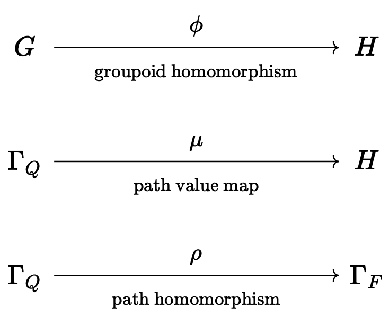## Graph covers

### Graph homomorphisms

Before we can define quiver covers, we'll briefly introduce graph covers and graph homomorphisms.

Formally, a (directed) graph cover $$\functionSignature{\function{\graphHomomorphism{ \pi }}}{\graph{G}}{\graph{H}}$$ is a surjective graph homomorphism $$\graphHomomorphism{ \pi }$$ between directed graphs $$\graph{G}$$ and $$\graph{H}$$. $$G$$ is then called a cover of $$\graph{H}$$, written $$\graph{G}\coversSymbol \graph{H}$$. We'll also write $$\graphCovering{\graphHomomorphism{ \pi }}{\graph{G}}{\graph{H}}$$ to explicitly indicate the homomorphism $$\graphHomomorphism{ \pi }$$ involved. But what is a graph homomorphism?

The homomorphism $$\graphHomomorphism{ \pi }$$ tells us how to "project" elements of $$\graph{G}$$ onto elements of $$\graph{H}$$, and consists of a map $$\functionSignature{\graphHomomorphism{ \pi _V}}{\vertexList(\graph{G})}{\vertexList(\graph{H})}$$ and a map $$\functionSignature{\graphHomomorphism{ \pi _E}}{\edgeList(\graph{G})}{\edgeList(\graph{H})}$$, subject to the fairly obvious compatibility condition that the vertices of the projection of an edge must be the projection of the vertices of that edge:

$\begin{array}{c} \graphHomomorphism{ \pi _E}(\de{\vert{g_1}}{\vert{g_2}}) = \de{\vert{h_1}}{\vert{h_2}}\\ \implies \\ \paren{\graphHomomorphism{ \pi _V}(\vert{g_1})\orSymbol \vert{h_1}}\andSymbol \paren{\graphHomomorphism{ \pi _V}(\vert{g_2})\orSymbol \vert{h_2}} \end{array}$

### Graph covers

A graph cover $$\graph{G}\coversSymbol \graph{H}$$ is a surjective graph homomorphism. Therefore one way we can depict a cover is by illustrating which vertices and edges of $$\graph{G}$$ cover which vertices and edges of $$\graph{H}$$. For small graphs, we will use additive color semantics to do this. In particular, if $$\graph{G}$$ has three vertices, we will depict them as $$\rform{\filledToken }$$, $$\gform{\filledToken }$$, $$\bform{\filledToken }$$. Then we'll show a vertex of $$\graph{H}$$ with a color that is the additive blend of the primary colors of its preimage under $$\graphHomomorphism{ \pi _V}$$. For example, $$\inverse{\graphHomomorphism{ \pi _V}}(\rgform{\filledToken }) = \list{\rform{\filledToken },\gform{\filledToken }}$$, $$\inverse{\graphHomomorphism{ \pi _V}}(\wcform{\filledToken }) = \list{\rform{\filledToken },\gform{\filledToken },\bform{\filledToken }}$$. Similarly we'll color the edges: $$\inverse{\graphHomomorphism{ \pi _E}}(\waform{\barToken }) = \list{\barToken ,\wcform{\barToken }}$$.

Let's look at very simple example undirected graph $$\graph{G}$$, which we'll call the partial triangle, and a particular $$\graph{H}$$ it covers: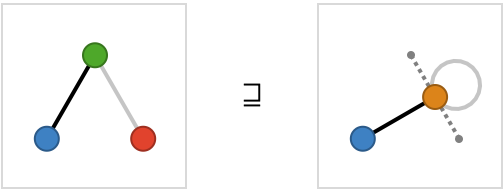The $$\rgform{\filledToken }$$ vertex of $$\graph{H}$$ is covered by the $$\rform{\filledToken }$$ and $$\gform{\filledToken }$$ vertices of $$\graph{G}$$, and the $$\bform{\filledToken }$$ vertex of $$\graph{H}$$ by the $$\bform{\filledToken }$$ vertex of $$\graph{G}$$. We’ll refer to these pre-images as contractions, so that we say $$\rgform{\filledToken }$$ is the contraction of $$\rform{\filledToken }$$ and $$\gform{\filledToken }$$. We've also shown dotted lines to their original positions to indicate that the $$\rform{\filledToken }$$ and $$\gform{\filledToken }$$ vertices were contracted together by this covering to form the $$\rgform{\filledToken }$$ vertex. The two edges are mapped uniquely here, and so remain colored $$\barToken$$ and $$\wcform{\barToken }$$, hence there are no edge contractions. However, the edge between $$\rform{\filledToken }$$ and $$\gform{\filledToken }$$ now become a self-loop on $$\rgform{\filledToken }$$.

### Covering partial order

Our partial triangle covers many smaller graphs. For finite graphs, it’s clear that the covered graph has the same or fewer vertices, so we can conclude that up to isomorphism there are only finitely many covered graphs of $$\graph{G}$$. Furthermore, if $$\graph{G}\coversSymbol \graph{H}\coversSymbol \graph{J}$$, it's clear that $$\graph{G}\coversSymbol \graph{J}$$, since the composition of two surjective homomorphisms is another. Therefore, covering forms a partial order on finite graphs, and we can depict this in the form of a larger vertical graph, with an edge between two graphs if the higher one strictly covers the lower one – in other words a graph is connected to one below it if it covers it, is not isomorphic, and the covering is not implied by transitivity.

Here is the covering partial order, or synonymously the contraction partial order, for our example: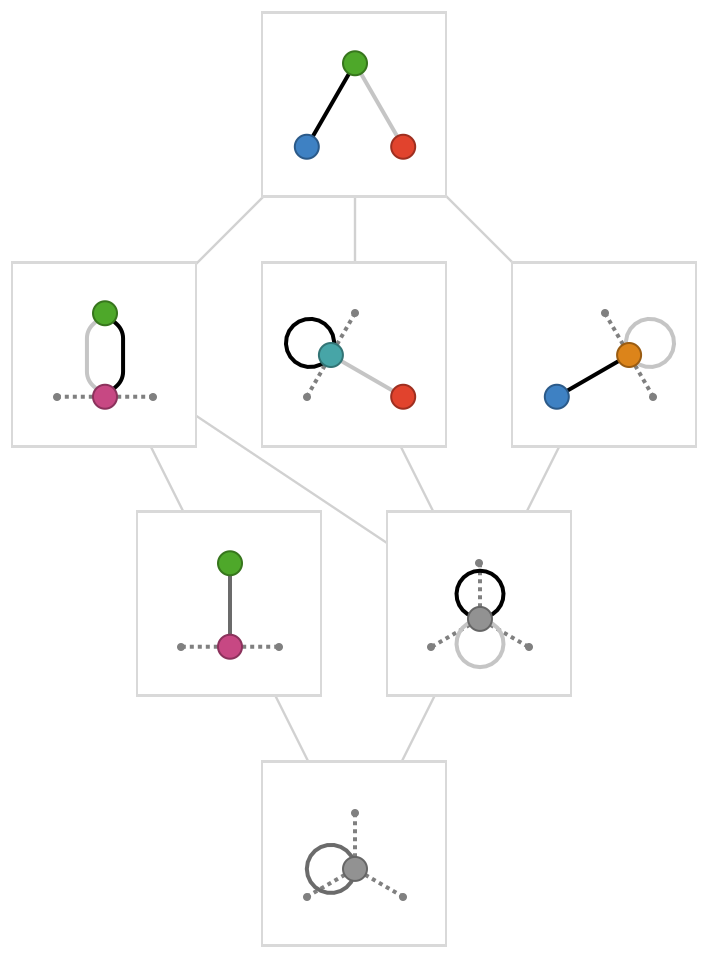### Square

Let's consider the covering partial order for a "partial square" with 4 vertices and 3 edges. To reduce uninteresting complexity, we will avoid the explicit contraction of edges, and perform these contractions "greedily" whenever multiple edges exist between two vertices as a result of a vertex contraction: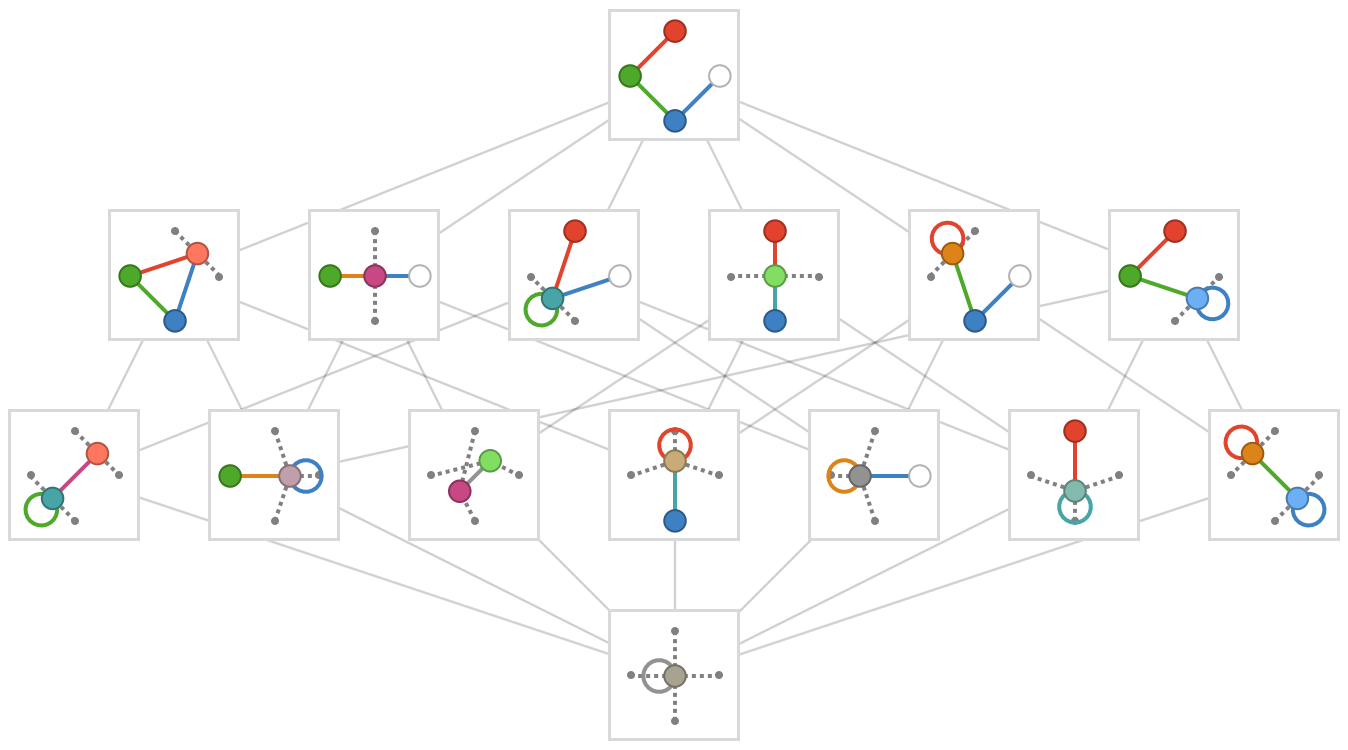Notice that as in the previous example, this partial order terminates in a single vertex and self-loop, the 1-graph, which is covered by every other graph. This single vertex and its self loop are gray, reflecting the fact that they are the color average of all of the original primary-colored vertices and edges.

## Quiver covers

What about quivers? How do we extend the notion of covering to the quiver setting? We saw that for graphs, we could effect any particular covering in a sequence of atomic steps, in which we contracted together pairs of vertices and edges. It turns out that this approach does not work for quivers, for the elementary reason that we can easily violate the local uniqueness property unless we perform multiple vertex contractions simultaneously.

#### Simultaneous contractions

Let's take a simple example to illustrate this idea. We'll focus on coverings of $$\bindCards{\subSize{\squareQuiver }{2}}{\rform{\card{r}},\bform{\card{b}}}$$: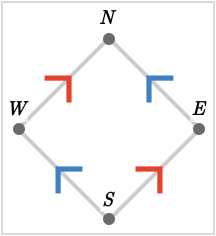Here we illustrate a particular graph covering: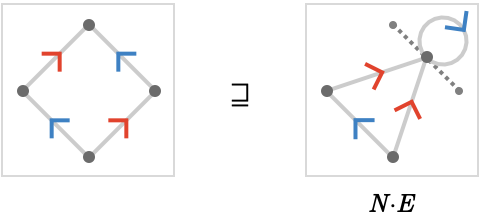Here, we have performed the contraction $$\contractionProduct{\vert{N}\contractionProductSymbol \vert{E}}$$, where $$\contractionProductSymbol$$ represents the contraction of two vertices. However the result is no longer a quiver, as the contracted vertex $$\contractionProduct{\vert{n}\contractionProductSymbol \vert{e}}$$ now has two incoming $$\rform{\card{r}}$$ cardinals. So while this is graph covering, it is not a quiver covering.

In contrast, here is a valid quiver covering, where we have performed the gluing $$\contractionSum{\contractionProduct{\vert{N}\contractionProductSymbol \vert{E}}\contractionSumSymbol \contractionProduct{\vert{S}\contractionProductSymbol \vert{W}}}$$, which consists of the simultaneous gluings $$\contractionProduct{\vert{N}\contractionProductSymbol \vert{E}}$$ and $$\contractionProduct{\vert{S}\contractionProductSymbol \vert{W}}$$: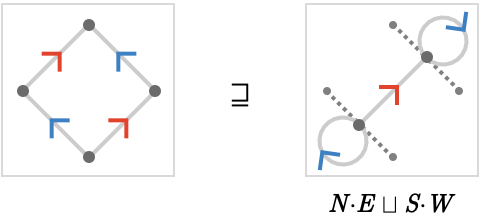You might object that there should be two edges with the cardinal $$\rform{\card{r}}$$ between the glued vertices $$\contractionProduct{\vert{N}\contractionProductSymbol \vert{E}}$$ and $$\contractionProduct{\vert{S}\contractionProductSymbol \vert{W}}$$. We simply define these two edges to be contracted together automatically and implicitly, and in fact we will only allow edge contractions that are precisely of this "deduplicating" form.

The key point here is that the requirement of preserving the local uniqueness property is what makes gluings such as $$\contractionProduct{\vert{N}\contractionProductSymbol \vert{E}}$$ impossible to perform in isolation: it is only when we perform $$\contractionSum{\contractionProduct{\vert{N}\contractionProductSymbol \vert{E}}\contractionSumSymbol \contractionProduct{\vert{S}\contractionProductSymbol \vert{W}}}$$ simultaneously that we preserve local uniqueness.

#### Contraction order

Let's compute the complete contraction order: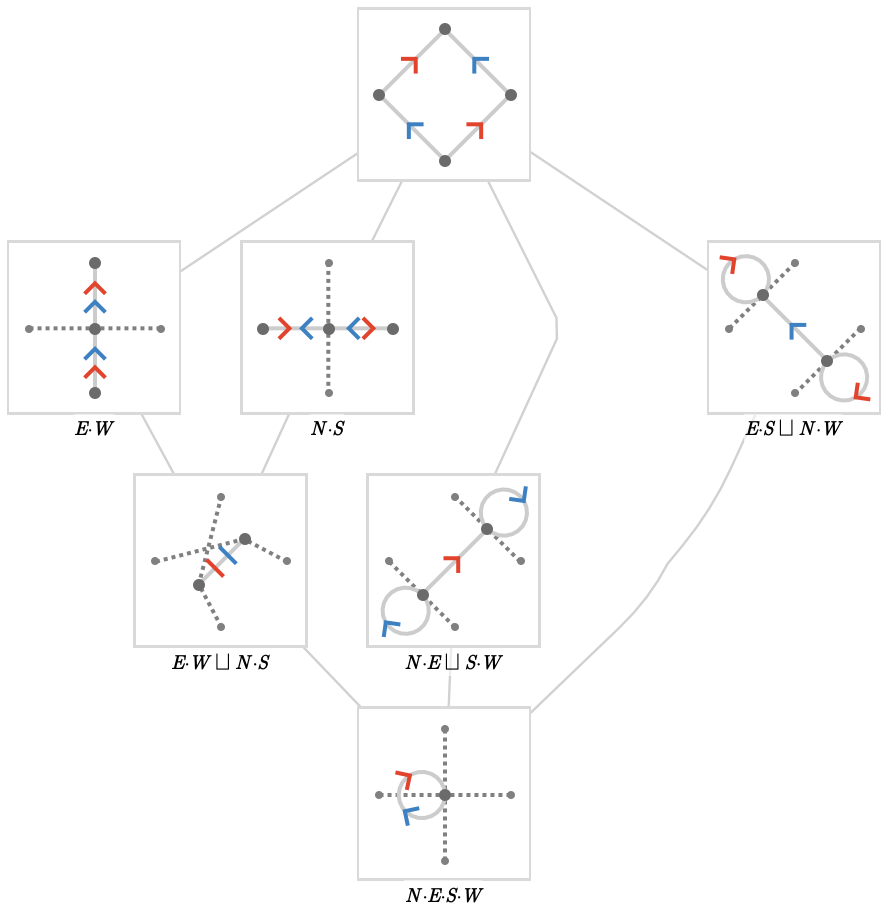In the section Contraction lattices we will examine this object from an order-theoretic point of view, establishing the structure of an order-theoretic lattice (which is a different usage of the word "lattice" than that in Lattice quivers).

## Path homomorphisms

Let's now turn to the question of the connection between path homomorphisms and quiver coverings.

Pictured below is a quiver $$\graph{G}$$, and 5 quivers that it covers: $$\graph{H_1},\graph{H_2},\graph{H_3},\graph{H_4},\graph{H_5}$$.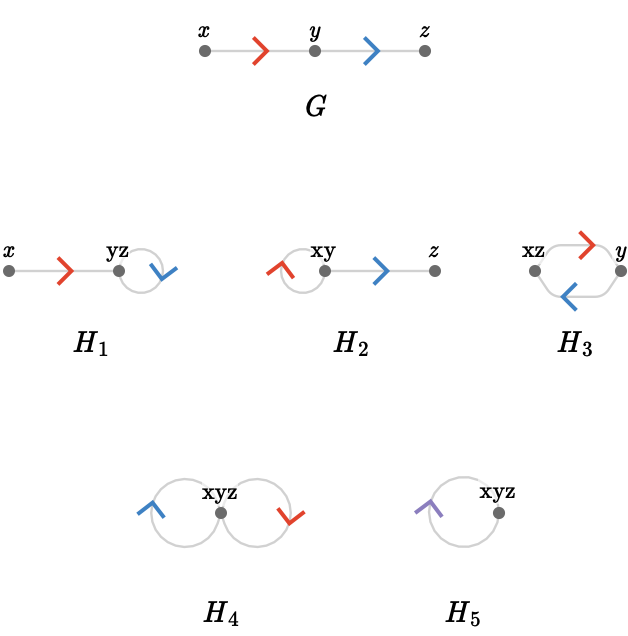These form a partial order as before, which looks like this: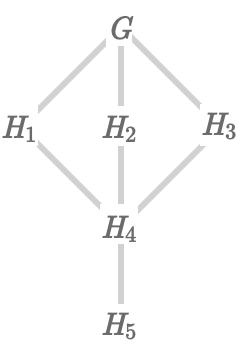Let's focus on a particular covering $$\graphCovering{\graphHomomorphism{ \pi }}{\quiver{G}}{\quiver{H_1}}$$, which is associated with some graph (quiver) homomorphism $$\graphHomomorphism{ \pi } = \tuple{\graphHomomorphism{ \pi _E},\graphHomomorphism{ \pi _V}}$$.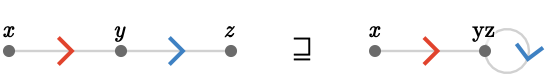An alternative way of expressing a quiver homomorphism is via a path homomorphism $$\functionSignature{\pathHomomorphism{ \rho }}{\pathGroupoid{\graph{G}}}{\pathGroupoid{\graph{H_1}}}$$. Why is this? I'll illustrate by providing a particular $$\pathHomomorphism{ \rho }$$ that corresponds to $$\graphHomomorphism{ \pi }$$, and illustrating its behavior with a path table: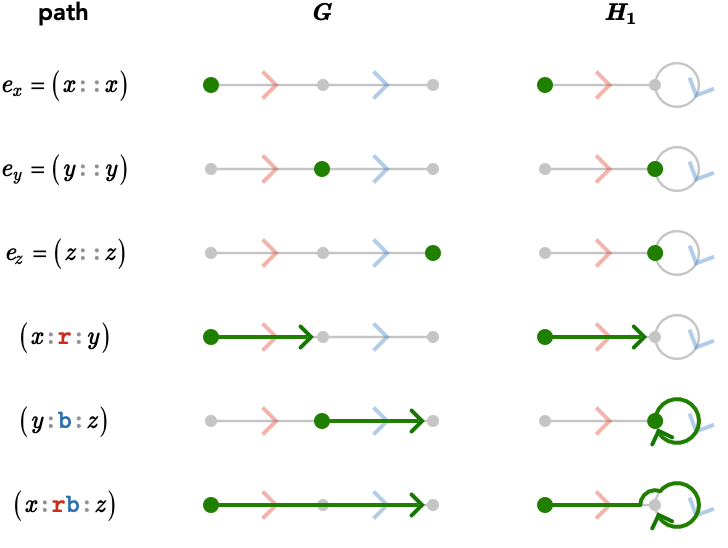As for any path homomorphism, empty paths are sent to empty paths, $$\pathHomomorphism{ \rho }(\paren{\pathWord{\vert{s}}{\emptyWord{}}{\vert{s}}}) = \paren{\pathWord{\vert{t}}{\emptyWord{}}{\vert{t}}}$$, giving us the vertex covering $$\graphHomomorphism{ \pi _V}(\vert{s}) = \vert{t}$$.

Additionally, this homomorphism is length-preserving: 1-paths are sent to 1-paths, $$\pathHomomorphism{ \rho }(\paren{\pathWord{\vert{u}}{\word{\card{c}}}{\vert{\primed{u}}}}) = \paren{\pathWord{\vert{v}}{\word{\card{c}}}{\vert{\primed{v}}}}$$, giving us the edge covering $$\graphHomomorphism{ \pi _E}(\tde{\vert{u}}{\primed{u}}{\card{c}}) = \tde{\vert{v}}{\primed{v}}{\card{c}}$$.

What about the compatibility condition between $$\graphHomomorphism{ \pi _E}$$ and $$\graphHomomorphism{ \pi _V}$$? This is a consequence (easily checked) of the ordinary compatibility condition of path homomorphisms: $$\pathHomomorphism{ \rho }(\pathCompose{\path{P_1}}{\path{P_2}}) = \pathCompose{\pathHomomorphism{ \rho }(\path{P_1})}{\pathHomomorphism{ \rho }(\path{P_2})}$$.

Ok, so coverings can be described by path homomorphisms. But are they uniquely so described? And do all path homomorphisms yield corresponding coverings?

#### Surjective path homomorphisms to coverings

To answer the latter question, let's answer a simpler question: can a path homomorphism send an empty path to a non-empty path? As we saw in Path homomorphisms, the answer is no. Therefore we can always obtain a vertex cover $$\graphHomomorphism{ \pi _V}$$ from a surjective path homomorphism, since this will guarantee that every vertex (= empty path) of the covered quiver has at least one corresponding vertex (= empty path) of the covering quiver.

Ok, so the $$\graphHomomorphism{ \pi _V}$$ story seems clear. To know that we can obtain an edge cover $$\graphHomomorphism{ \pi _E}$$ from $$\pathHomomorphism{ \rho }$$, let's imagine how it could go wrong: can a 1-path can be sent to e.g. a 2-path? Again, we saw examples of path-lengthening homomorphisms already in [[[Path homomorphisms]], but here is a path table showing a simple example: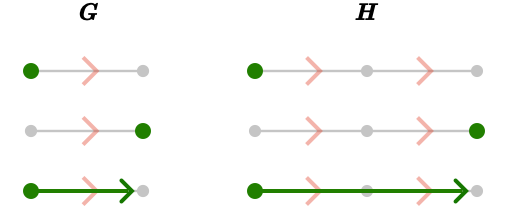This path homomorphism is not surjective, of course: the 1-paths in $$\quiver{H}$$ have no preimage under $$\pathHomomorphism{ \rho }$$. However, we can construct a more elaborate surjective homomorphism that is still path lengthening? Here is such an example: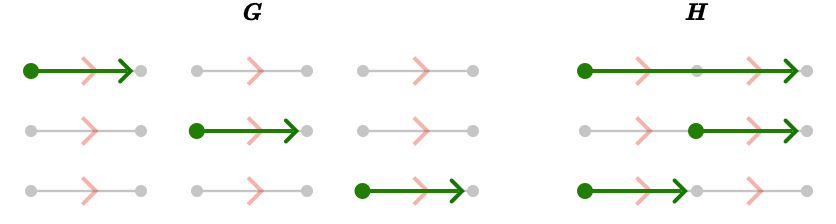Clearly, if the covering quiver is disconnected we have considerable freedom in defining path homomorphisms.

Another counterexample to the hypothesis that surjective path homomorphisms yield coverings is given by the following surjective path shortening homomorphism: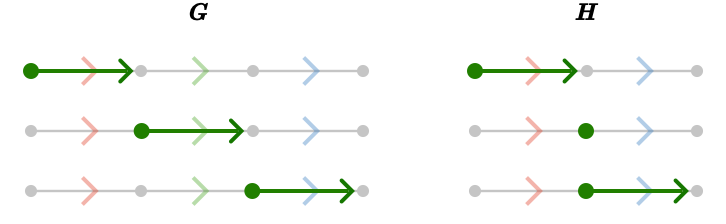Here, in moving from $$\quiver{G}$$ to $$\quiver{H}$$, we have contracted the edge labeled with cardinal $$\gform{\card{g}}$$, effectively deleting that cardinal from the path word of any path that passes through it. This is not a covering, because a covering must send edges of $$\quiver{G}$$ to edges of $$\quiver{H}$$.

The tentative answer then seems to be that without additional restrictions, a surjective path homomorphism does not automatically yield a covering. But the requirement we need is easily guessed: we must have a length-preserving path homomorphism, since this defines a unique (oriented) edge in the covered graph for every edge in the covering graph in a compatible way.

#### Coverings to surjective path homomorphisms

Does a covering $$\graphCovering{\function{ \pi }}{\quiver{G}}{\quiver{H}}$$ induce a unique path homomorphism $$\pathHomomorphism{ \rho }$$? The answer here is more satisfying: yes! The reason of course is simple: the data of $$\graphHomomorphism{ \pi _E}$$ and $$\graphHomomorphism{ \pi _V}$$ does uniquely determine the behavior of $$\pathHomomorphism{ \rho }$$ on 0-paths and 1-paths. And since all longer paths in $$\graph{G}$$ can be constructed from compositions of 1-paths, and composition must be preserved by $$\pathHomomorphism{ \rho }$$, we have no choice in how to define $$\pathHomomorphism{ \rho }$$ on such longer paths. Hence, a covering yields (or generates) a path homomorphism.

We write this as $$\quiverCovering{\pathHomomorphism{ \gamma }}{\quiver{G}}{\quiver{H}}$$, and call $$\pathHomomorphism{ \gamma }$$ a covering homomorphism.

## Path quiver homomorphism

A more subtle kind of path homomorphism has been latent since we introduced path quivers: the path homomorphisms from a quiver to its forward path quiver. To construct these, we need to map every path in the quiver to a path in the path quiver (a “path of paths”). Let’s start with the easy part: if the path in the base quiver starts at the origin, we form the path in the path quiver by taking the sequence of extensions that extend the empty path into the full path: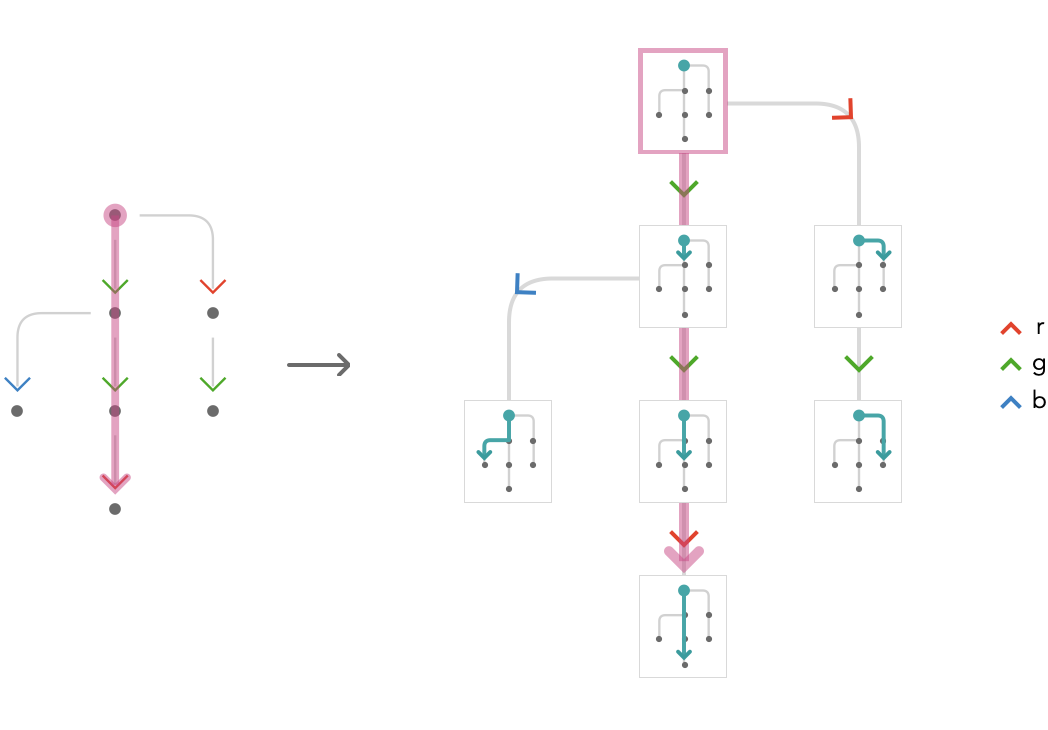In this example, we’ve sent a path in the base quiver with path word $$\word{\gform{\card{g}}}{\gform{\card{g}}}{\rform{\card{r}}}$$ to a path in the path quiver with path word $$\word{\gform{\card{g}}}{\gform{\card{g}}}{\rform{\card{r}}}$$.

But to define a path homomorphism, we also need to figure out where to send paths which do not start at the origin. For the tree quiver example, this is straightforward: for a path $$\path{P}$$ in the base quiver starting at $$\vert{t} \neq \vert{o}$$, we choose a vertex in the path quiver corresponding to the path taking us from the origin $$\vert{o}$$ to $$\vert{t}$$, then use the path word as before: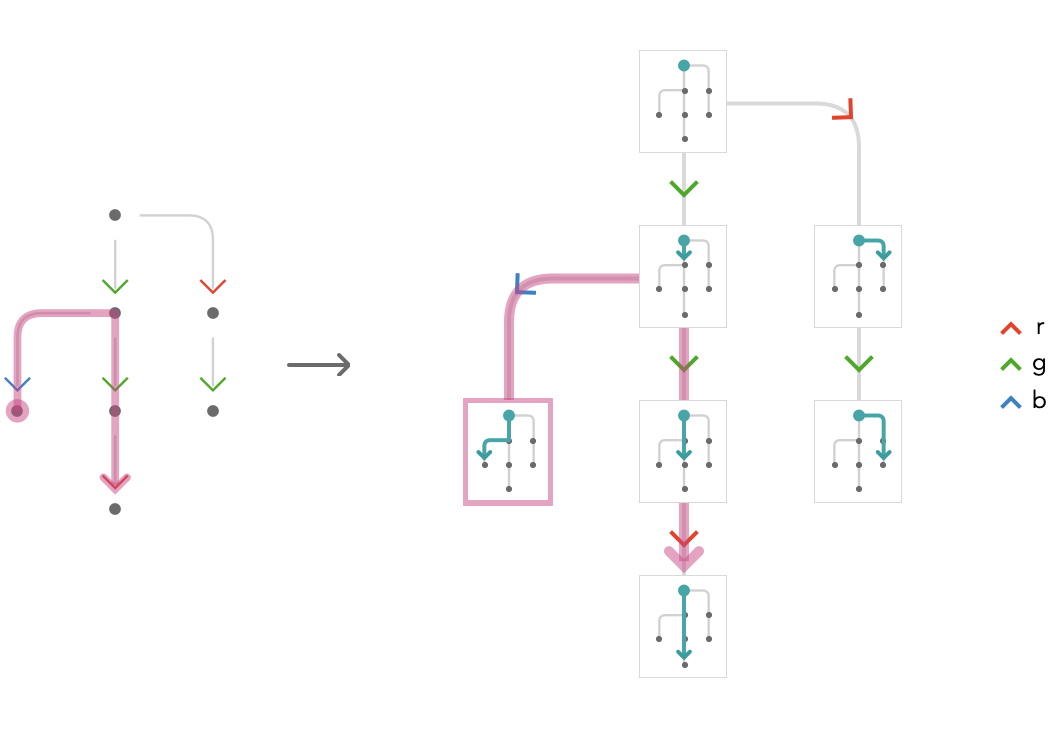In the case of trees the of this path homomorphism from a quiver to its path quiver is trivial, because the path quivers of trees are already isomorphic to them. But it turns out a related construction will work for all finite connected quivers, not just trees. Let’s look at a simple base quiver that is not a tree: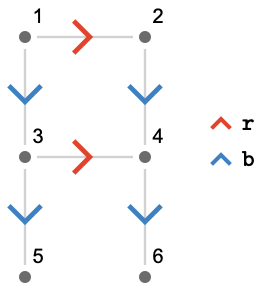Because there is a cycle $$\paren{\pathWord{\vert{1}}{\word{\rform{\card{r}}}{\bform{\card{b}}}{\rform{\ncard{r}}}{\bform{\ncard{b}}}}{\vert{1}}}$$, the path quiver will be infinite, so we’ll only draw the subgraph of the path quiver in which this cycle is “wound” a maximum of one time. Choosing the origin as $$\vert{1}$$, we again have no problem with the “easy” part of where to send paths starting at the origin, since they just have the same cardinal word. For example, the path $$\paren{\pathWord{\vert{1}}{\word{\bform{\card{b}}}{\rform{\card{r}}}{\bform{\card{b}}}}{\vert{6}}}$$: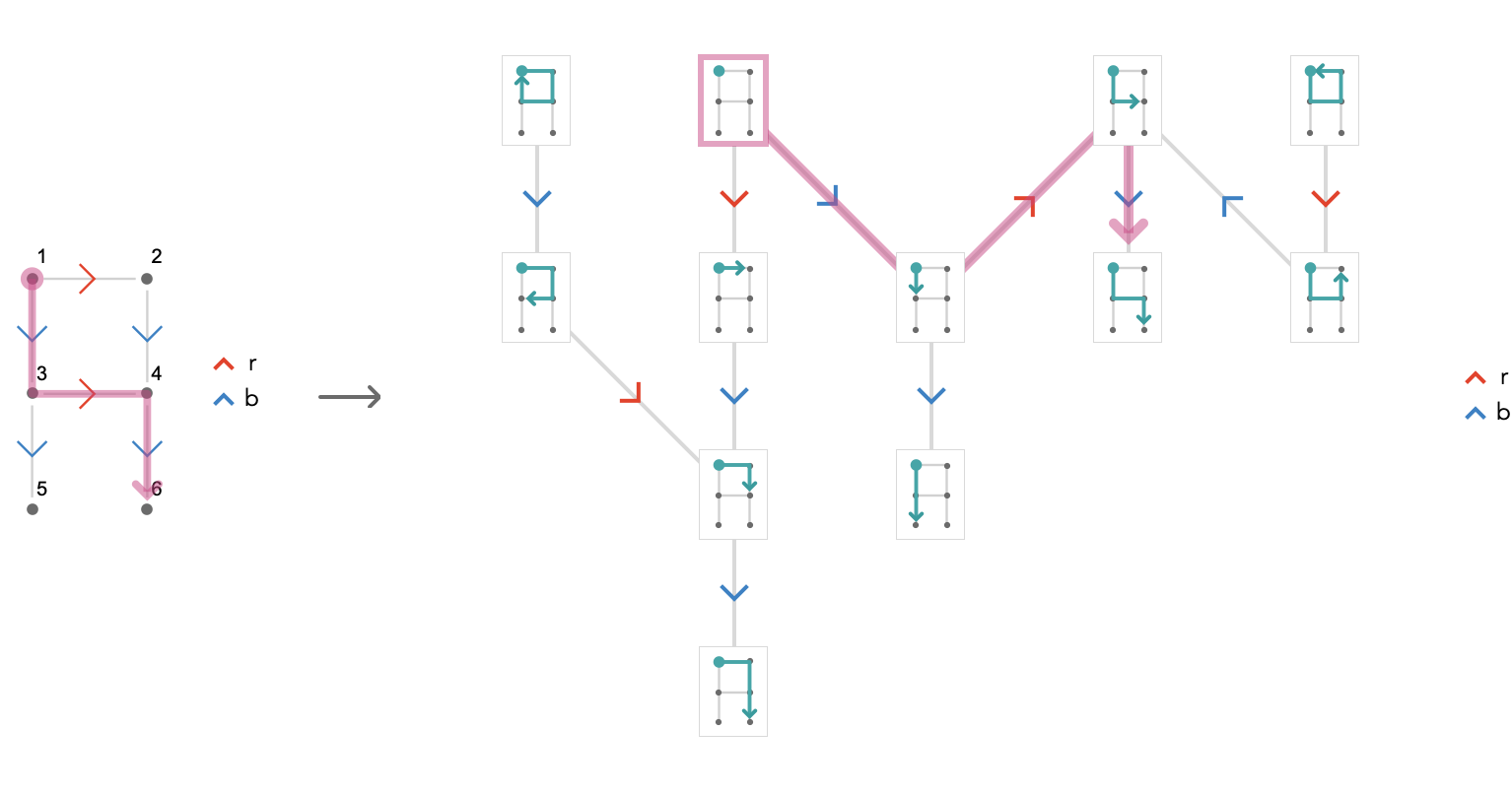The “hard” part consists of dealing with paths like this: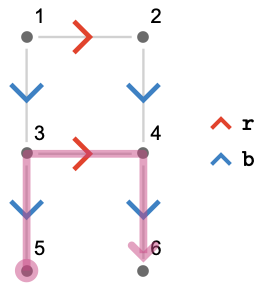To figure out where our path homomorphism $$\pathHomomorphism{ \rho }$$ sends this path $$\path{P} = \paren{\pathWord{\vert{5}}{\word{\bform{\ncard{b}}}{\rform{\card{r}}}{\bform{\card{b}}}}{\vert{6}}}$$ must first choose a vertex $$\elemOf{\vert{t}}{\forwardPathQuiver{\quiver{Q}}{\vert{1}}}$$ that corresponds to the tail vertex of $$\path{P}$$, which is $$\vert{5}$$. Now $$\pathHomomorphism{ \rho }(\vert{5})$$ is a path with head vertex $$\vert{5}$$. One we’ve chosen $$\pathHomomorphism{ \rho }(\vert{t})$$, there is nothing more to do, since the path word of $$\path{P}$$ gives the path word of $$\pathHomomorphism{ \rho }(\path{P})$$. However, whatever choice we make for $$\pathHomomorphism{ \rho }(\vert{5})$$ must be compatible with other choices we make for other vertices in $$\quiver{Q}$$. Luckily, this isn’t as tricky as it sounds. All we need to do is choose a spanning tree of the base quiver.## Fundamental quiver

We now return to the situation of a lattice quiver $$\quiver{Q}$$ generated by a fundamental quiver $$\bindCards{\quiver{F}}{\card{\card{c}_1},\card{\ellipsis },\card{\card{c}_{\sym{i}}}}$$ with the representation associated with a cardinal valuation $$\functionSignature{\groupoidFunction{ \phi }}{\cardinalList(\quiver{Q})}{\group{G}}$$. That $$\quiver{Q}$$ is generated by $$\quiver{F}$$ is the statement:

$\quiver{Q} = \compactQuotient{\quiver{F}}{\vert{v}}{\groupoidFunction{ \phi }}$

An important fact about lattice quivers is they cover their fundamental quivers: $$\graphCovering{\graphHomomorphism{ \pi }}{\quiver{Q}}{\quiver{F}}$$. To this covering is associated a path homomorphism $$\pathHomomorphism{ \rho }$$, which we use to define the covering as follows:

\begin{aligned} \pathHomomorphism{ \rho }(\pathWord{\primed{\vert{t}}}{\wordSymbol{W}}{\primed{\vert{h}}})&\defEqualSymbol \pathWord{\vert{t}}{\wordSymbol{W}}{\vert{h}}\\ \primed{\vert{t}}&\defEqualSymbol \tuple{\vert{t},\groupoidElement{g}_1}\\ \primed{\vert{h}}&\defEqualSymbol \tuple{\vert{h},\groupoidElement{g}_2}\end{aligned}

Here we use the fact that $$\elemOf{\primed{\vert{v}}}{\vertexList(\quiver{Q})}$$ are precisely pairs $$\tuple{\vert{v},\groupoidElement{g}}$$, where $$\elemOf{\vert{t}}{\vertexList(\quiver{F})}$$ and $$\groupoidElement{g}$$ are elements of the group associated with the cardinal valuation $$\groupoidFunction{ \phi }$$.